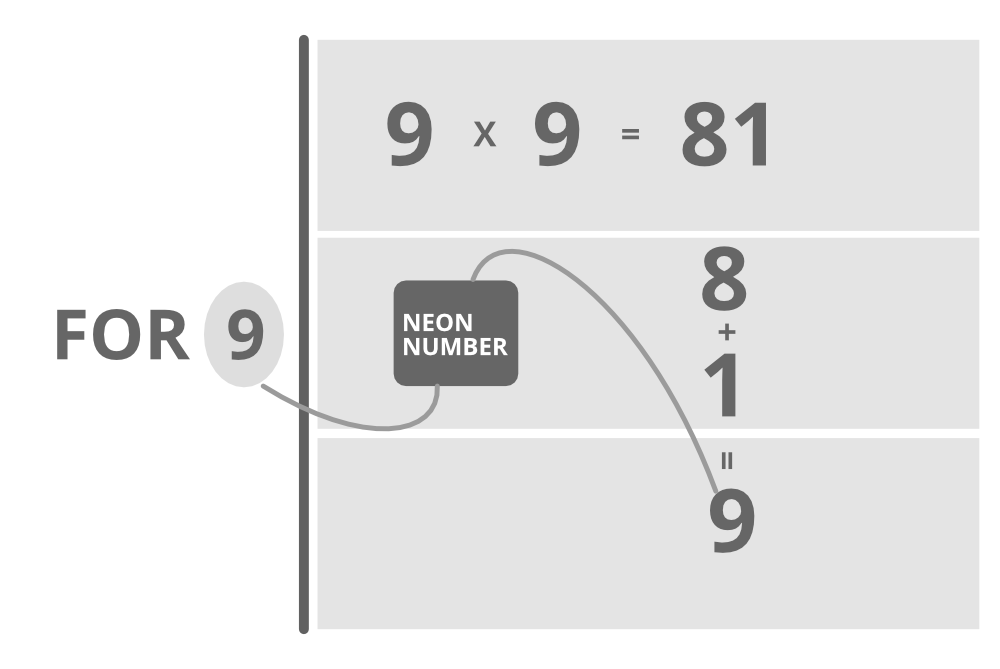Related Articles
Java Program to Check If a Number is Neon Number or Not
• Difficulty Level : Medium
• Last Updated : 13 Jan, 2021

A neon number is a number where the sum of digits of the square of the number is equal to the number. The task is to check and print neon numbers in a range.Illustration:

```Case 1:

Input  : 9
Output : Given number  9 is Neon number

Explanation : square of 9=9*9=81;
sum of digit of square : 8+1=9(which is equal to given number)

Case 2:

Input : 8
Output : Given number is not a Neon number

Explanation : square of 8=8*8=64
sum of digit of square : 6+4=10(which is not equal to given number)```

Algorithm :

1. First, find the square of the given number.
2. Find the sum of the digit of the square by using a loop.

3. The condition checksum is equal to the given number
1. Return true
2. Else return false.
```Pseudo code : Square =n*n;
while(square>0)
{
int r=square%10;
sum+=r;
square=square/10;
}```

Example:

## Java

 `// Java Program to Check If a Number is Neon number or not`` ` `// Importing java input/output library``import` `java.io.*;`` ` `class` `GFG {`` ` `    ``// Method to check whether number is neon or not``    ``// Boolean type``    ``public` `static` `boolean` `checkNeon(``int` `n)``    ``{``        ``// squarring the number to be checked``        ``int` `square = n * n;`` ` `        ``// Initializing current sum to 0``        ``int` `sum = ``0``;`` ` `        ``// If product is positive``        ``while` `(square > ``0``) {`` ` `            ``// Step 1: Find remainder``            ``int` `r = square % ``10``;`` ` `            ``// Add remainder to the current sum``            ``sum += r;`` ` `            ``// Drop last digit of the product``            ``// and store the number``            ``square = square / ``10``;``        ``}`` ` `        ``// Condition check``        ``// Sum of digits of number obtained is``        ``// equal to original number``        ``if` `(sum == n)`` ` `            ``// number is neon``            ``return` `true``;``        ``else`` ` `            ``// numbe is not neon``            ``return` `false``;``    ``}`` ` `    ``// Main driver method``    ``public` `static` `void` `main(String[] args)``    ``{``        ``// Custom input``        ``int` `n = ``9``;`` ` `        ``// Calling above functon to check custom number or``        ``// if user entered number via Scanner class``        ``if` `(checkNeon(n))`` ` `            ``// Print number considered is neon``            ``System.out.println(``"Given number "` `+ n``                               ``+ ``" is Neon number"``);``        ``else`` ` `            ``// Print number considered is not neon``            ``System.out.println(``"Given number "` `+ n``                               ``+ ``" is not a Neon number"``);``    ``}``}`
Output
`Given number 9 is Neon number`

Time Complexit: O(l) where l is the number of the digit in the square of the given number

Attention reader! Don’t stop learning now. Get hold of all the important Java Foundation and Collections concepts with the Fundamentals of Java and Java Collections Course at a student-friendly price and become industry ready. To complete your preparation from learning a language to DS Algo and many more,  please refer Complete Interview Preparation Course.

My Personal Notes arrow_drop_up